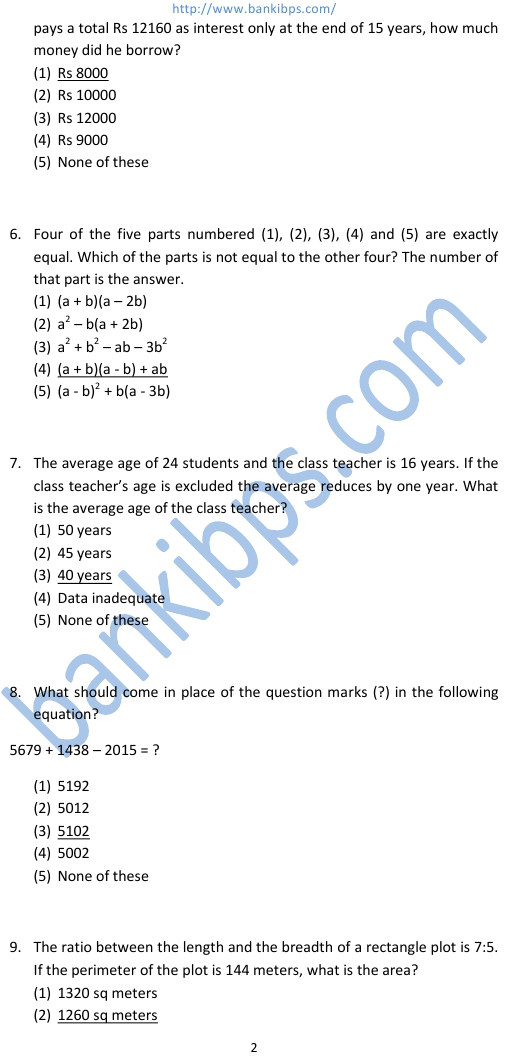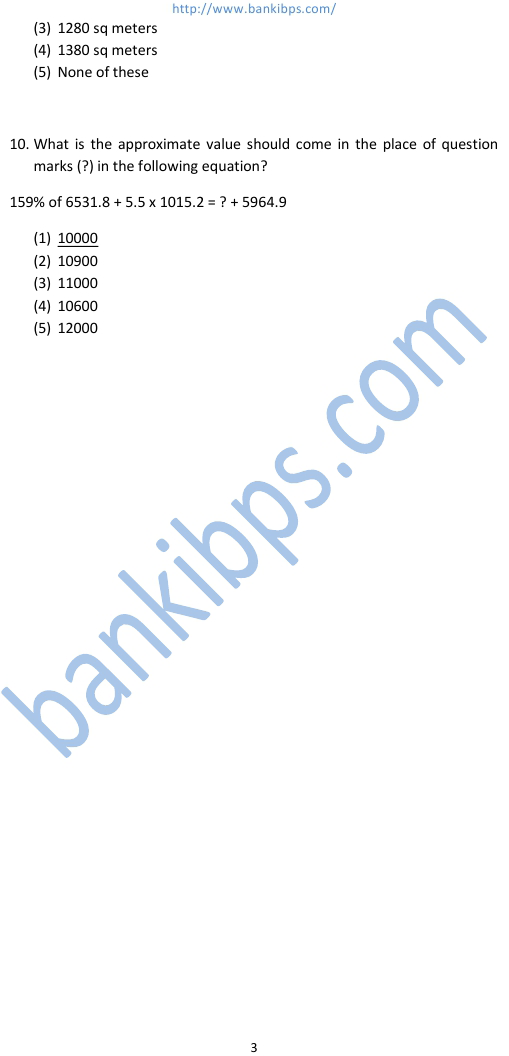# IBPS RRB CWE Previous Question Papers

1. What is the approximate value should come in the place of (?) marks in the following equation? 6.595 x 1048 + 2568.34 – 1708.34 = ? (1) 600 (2) 12000 (3) 10000 (4) 8000 (5) 9000 ibps rrb cwe previous question papers 2. Four-fifth of three-eighths of a number is 24. What is 250 percent of that number? (1) 100 (2) 160 (3) 120 (4) 200 (5) None of these 3. The average of 17 numbers is 45. The average of first 9 of these numbers is 51 and the last 9 of these numbers is 36. What is the ninth number? (1) 14 (2) 16 (3) 22 (4) 20 (5) None of these 4. Four of the five parts numbered (1), (2), (3), (4) and (5) are exactly equal. Which of the parts is not equal to the other four? The number of that part is the answer. (1) 16.80 x 4.50 + 4.4 (2) 1600 ÷ 40 + 16 x 2.5 (3) 5.5 x 8.4 + 34.6 (4) 1620 ÷ 20 -1 (5) 1856.95 – 1690.65 – 96.3
Practice Exercise - 293 [ Aptitude ]## ibps rrb cwe previous question papers

### ibps cwe for rrb

#### ibps rrb cwe exam

##### ibps cwe rrb 2012 model papers
###### ibps cwe rrb model papers
ibps cwe for rrb. ibps rrb cwe exam. ibps cwe rrb 2012 model papers. ibps cwe rrb model papers. rrb question papers and answers. rrb question and answer. rrb questions and answers. ibps rrb model question paper. ibps rrb exam model question paper. ibps rrb model question papers. rrb exam model question paper answer. rrb exams model question papers.